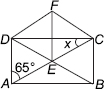# User Forum

Subject :IMO    Class : Class 7

In the figure, ABCD is a rectangle, ΔCEF is an equilateral triangle. Find x.A 25º
B 30º
C 20º
D 50°

given that Î CEF is an equilateral triangle, each of its angles are 60Â° and also shown in the figure that DC bisects angle C, so angle x should be 30Âº

Class : Class 7